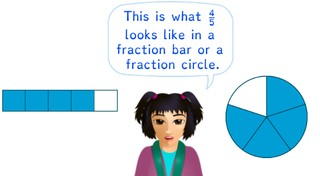Writing a fraction in a fraction bar

# Writing a fraction in a fraction bar

Students learn to write a fraction in a fraction bar.8,000 schools use Gynzy92,000 teachers use Gynzy1,600,000 students use Gynzy

## General

The students learn how to write a fraction in a fraction bar or a fraction circle.

## Standards

CCSS.Math.Content.3.NF.A.1

## Learning objective

Students will be able to write a fraction in a fraction bar or in a fraction circle.

## Introduction

Have the students listen to the sound fragments. The need to write down the fraction that they hear. Have them hold up their answers, so that you can check whether they have written the correct fraction. After all of the speakers have been played, have the students determine which image matches each of the fractions. Drag each image to the matching fraction.

## Instruction

Explain that each fraction consists of a numerator and a denominator. After this you explain through an example how you write a fraction in a fraction bar. First see how many parts you should divide the bar up into. The denominator tells this. Then you divide the bar up into this number of parts. After this you see how many of the parts should be colored in. This is given by the numerator. Then you can color in the correct number of parts. Next you show an image with an accompanying fraction. The students must fill in the fraction bar up until the given fraction. Click on the parts in the bar, so that they are colored in. The students must also fill in a fraction bar according to a fraction, for which the bar is not yet divided into the correct number of parts. Ask the students how many parts the bar should be divided up into, and click on the + or - to add or remove divisions in the bar. After this you show the bars without visual support. The students must determine how much of the bar needs to be colored in. Click on the parts, so that the bar is colored in. Next you explain through an example how you can show a fraction in a fraction circle. First see how many parts you need to divide the circle into. The denominator tells this. Then you divide the fraction circle into that number of parts. After this you see how many parts need to be colored in. The numerator tells this. Then you can color in the correct number of parts. Next you show an example with an accompanying fraction. The students must fill in a fraction circle until the given fraction. Click on the parts of the fraction circle, so that it is colored according to the fraction. The students must also fill in a fraction circle for which the circle is not yet divided into the correct number of parts. Click on the + or - to add or remove parts to the fraction circle. After this you show fraction circles without visual support. The students must determine until which part the fraction circle must be colored in. Click on this part, so that the correct amount is colored in. There are also questions in which a fraction circle is not yet divided into the correct number of parts.

To check whether the students can write fractions in a fraction bar or a fraction circle, you can ask the following questions:
- Which part of the fraction tells how many parts of fraction bar or circle should be colored in: the numerator or the denominator?
- Which part of the fraction tells how many parts the fraction bar or circle should be divided up into: the numerator or the denominator?

## Quiz

The students test their understanding of writing a fraction in a fraction bar through ten exercises. In each of these exercises, they must determine how much of a fraction bar should be filled in, based on a given written fraction. Some of the fraction bars have already been divided up into parts, and for others the students must also divide the fraction bar into the correct number of parts.

## Closing

You discuss once again with the students that it is useful to be able to write a fraction in a fraction bar or circle, so that you can show which division is given by a fraction. Next you have the students work in pairs. One student names a fraction. Their partner draws this fraction in a fraction bar. After this they trade roles.
After this the students can spin two wheels the numbers form a fraction. The smallest number is the numerator and the largest number is the denominator. Next you have the students draw this fraction correctly in the fraction circle.

## Teaching tips

Students that have difficulty with writing a fraction can first practice with reading fractions. Emphasize that the number on top of the fraction tells how many parts should be colored in. The bottom number tells how many parts the bar or circle is divided up into. Next they can practice giving a fraction by drawing it first.

### The online teaching platform for interactive whiteboards and displays in schools

• Save time building lessons

• Manage the classroom more efficiently

• Increase student engagement